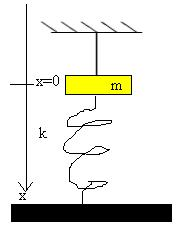# Harmonic oscillator

## Homework Statement

a mass is placed on a loose spring and connected to the ceiling. the spring is connected to the floor in t=0 the wire is cut
a. find the equation of the motion
b. solve the equation under the initial conditions due to the question## Homework Equations

## \sum F=ma
##
## x(t)=Asin(\omega t + \phi ) ##

## The Attempt at a Solution

a. due to the 2 law of newton: ## \sum F=ma_x
##
## mg-kx=ma ##
##
mg-kx=m\ddot{x}
\\
\ddot{x}=g-\frac{k}{m}x ##

b. first I'll find the point equilibrium
##
c-kx=ma
\\
c-kx=m\cdot 0
\\
x_0=\frac{c}{k}

##

then I'll define ## y=x-x_0 ##

How do I go from here?
thanks

TSny
Homework Helper
Gold Member
##\ddot{x}=g-\frac{k}{m}x ##
OK

##x_0=\frac{c}{k}##
What does c stand for?

then I'll define ## y=x-x_0 ##

How do I go from here?
Rewrite the differential equation in terms of y instead of x.

•quas
As TSny hints, you tripped finding x0. Think again what condition will be true at equilibrium and define x0 again. There won't be an unknown c.

•quas
OK

What does c stand for?

Rewrite the differential equation in terms of y instead of x.
sorry I meant ## x_0 = \frac{mg}{k}##
ok and then ## \ddot{y}=g-\frac{k}{m}y ## ?

Last edited:
BvU
Homework Helper
##
\ddot{y}=g-\frac{k}{m}y## can't be right. Compare with ##
\ddot{x}=g-\frac{k}{m}x##

##
\ddot{y}=g-\frac{k}{m}y## can't be right. Compare with ##
\ddot{x}=g-\frac{k}{m}x##
I might have a barrier but I do not understand how to build a differential equation for y,,,,
I understand that ## \dot{x}=\frac{dx}{dt}=\frac{dy}{dt}=\dot{y} \\ a=\ddot{x}=\frac{d^2x}{dt^2}=\frac{d^2y}{dt^2}=\ddot{y} ##

##
\ddot{y}=g-\frac{k}{m}y## can't be right. Compare with ##
\ddot{x}=g-\frac{k}{m}x##
??? I must be thick this morning, but if positive y is down that looks ok to me.

??? I must be thick this morning, but if positive y is down that looks ok to me.

Oh, good grief. It took me a minute!!

I might have a barrier but I do not understand how to build a differential equation for y,,,,
I understand that ## \dot{x}=\frac{dx}{dt}=\frac{dy}{dt}=\dot{y} \\ a=\ddot{x}=\frac{d^2x}{dt^2}=\frac{d^2y}{dt^2}=\ddot{y} ##

Much later after it stops oscillating and y is y0 what is the acceleration? g?

BvU
Simple: the second derivatives on the left are equal alright. But you need to substitute your expression for y in terms of x. Not just y = x, but: ...